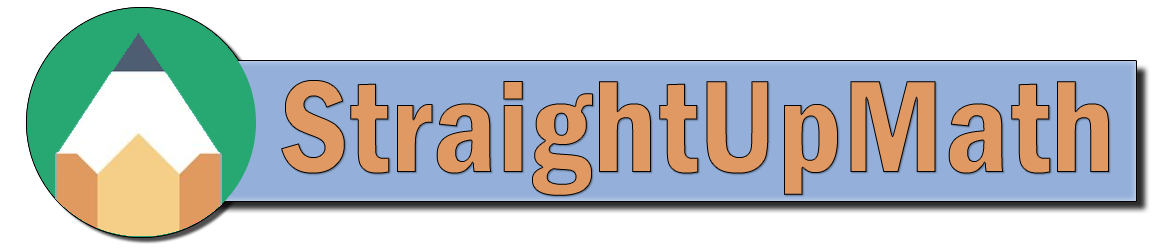Fractions - Lowest Common Denominator

Sorry, no video for this problem at this time.

## Practice

Find the lowest common denominator for the fractions given below.
a)
1
4
and
2
10

LCD = 20

b)
1
3
and
1
7

LCD = 21

c)
3
7
and
5
8

LCD = 56

d)
2
4
and
3
8

LCD = 8

e)
6
9
and
4
12

LCD = 36

f)
1
2
and
2
4

LCD = 4